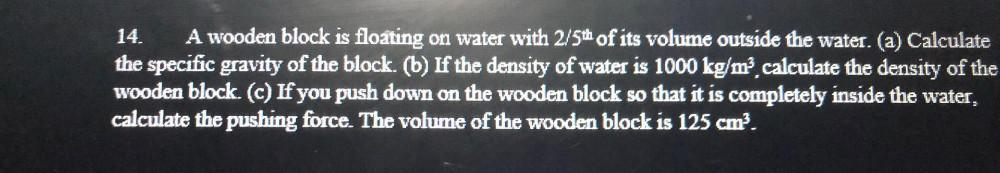Question:

# A wooden block is floating on water with 2/5th of its volume outside the water. (a) Calculate the specific gravity of the block. (b) If the density of water is 1000 kg/m³, calculate the density of thA wooden block is floating on water with 2/5th of its volume outside the water. (a) Calculate the specific gravity of the block. (b) If the density of water is 1000 kg/m³, calculate the density of the wooden block. (c) If you push down on the wooden block so that it is completely inside the water, calculate the pushing force. The volume of the wooden block is 125 cm³.